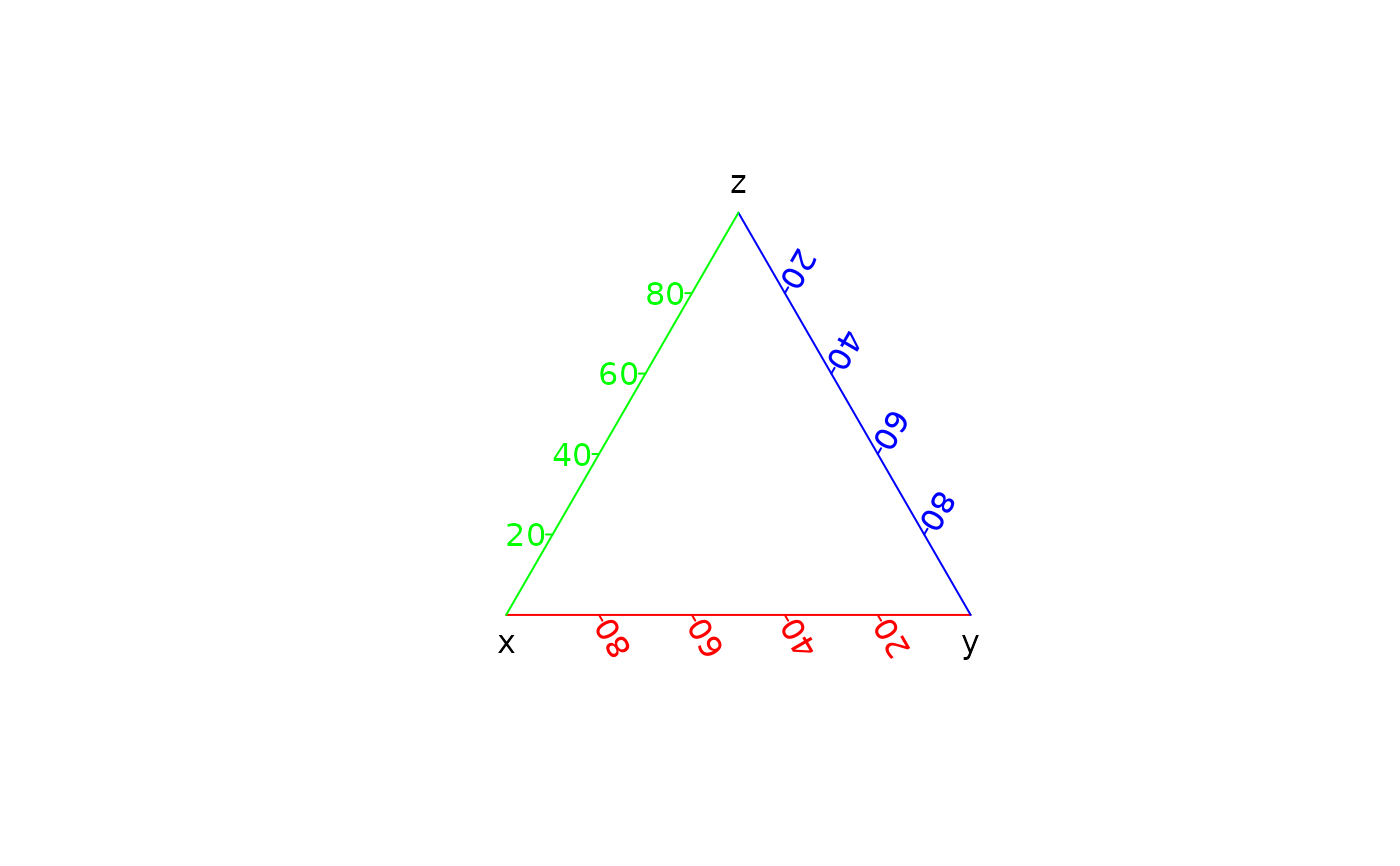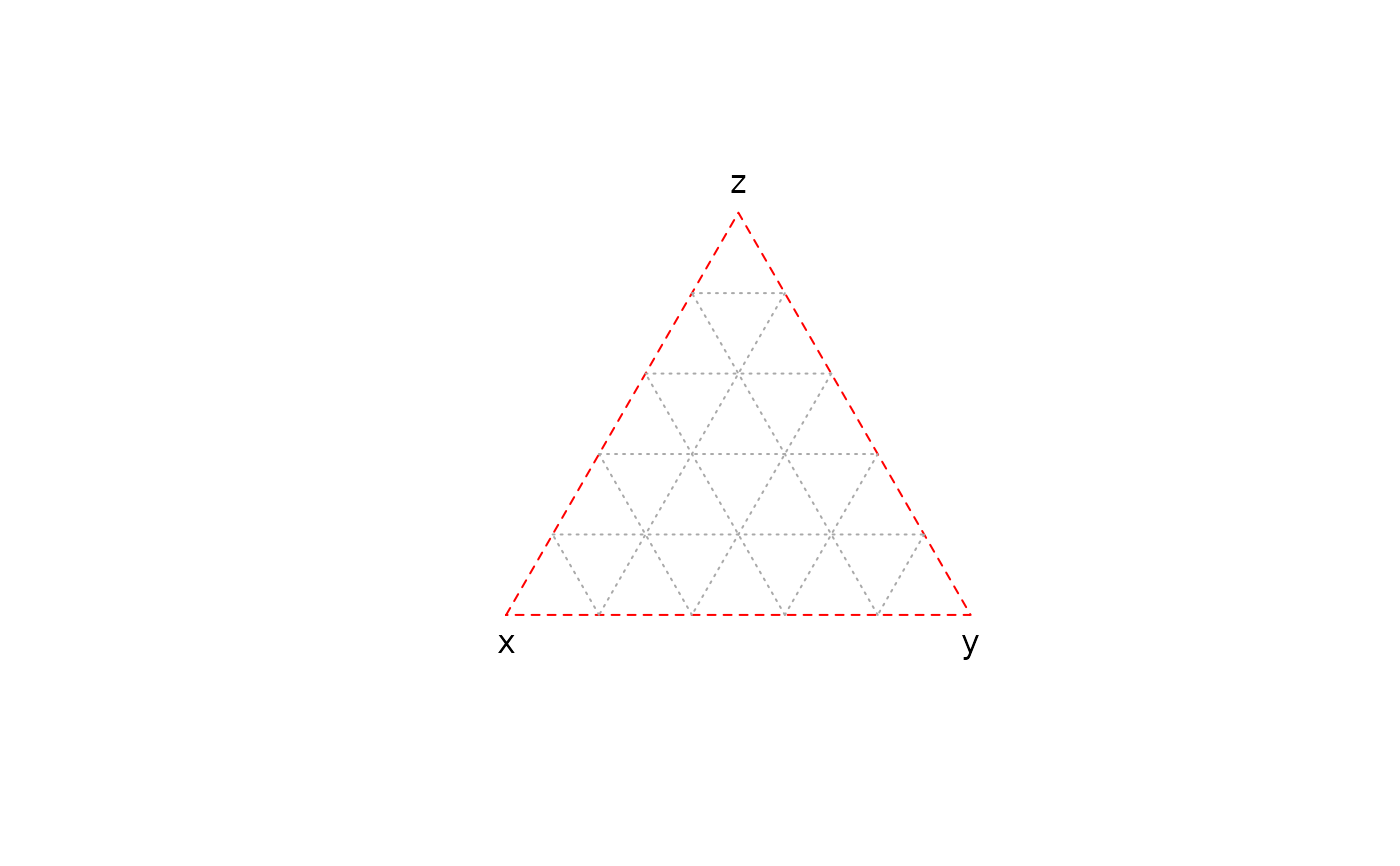Adds an axis to the current plot.

## Usage

ternary_axis(
side,
at = NULL,
labels = TRUE,
tick = TRUE,
font = NA,
lty = "solid",
lwd = 1,
lwd.ticks = lwd,
col = NULL,
col.ticks = NULL,
...
)

## Arguments

side

An integer specifying which side of the plot the axis is to be drawn on. The axis is placed as follows: 1=below, 2=right and 3=left.

at

A numeric vector giving the points at which tick-marks are to be drawn.

labels

A logical scalar specifying whether (numerical) annotations are to be made at the tickmarks, or a character vector of labels to be placed at the tickpoints. If this is not logical, at should also be supplied and of the same length.

tick

A logical scalar: should tickmarks and an axis line be drawn?

font

font for text. Defaults to par("font.axis").

lty

A character string or numeric value specifying the line type for both the axis line and the tick marks.

lwd, lwd.ticks

A non-negative numeric value specifying the line widths for the axis line and the tick marks.

col, col.ticks

Colors for the axis line and the tick marks respectively. Defaults to par("col.axis").

...

Other graphical parameters may also be passed as arguments to this function, particularly, cex.axis, col.axis and font.axis for axis annotation.

## Value

ternary_axis() is called it for its side-effects.

Other graphical elements: ternary_box(), ternary_grid(), ternary_pairs(), ternary_plot(), ternary_title()

N. Frerebeau

## Examples

## Add axis
ternary_plot(NULL, axes = FALSE)
ternary_axis(side = 1, col = "red")
ternary_axis(side = 2, col = "blue")
ternary_axis(side = 3, col = "green")